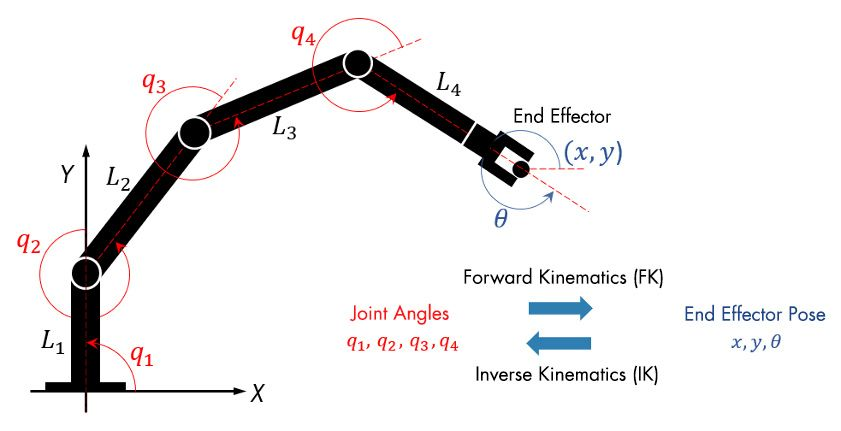# Inverse Kinematics

## Inverse kinematics (IK) algorithm design with MATLAB and Simulink

Kinematics is the study of motion without considering the cause of the motion, such as forces and torques. Inverse kinematics is the use of kinematic equations to determine the motion of a robot to reach a desired position. For example, to perform automated bin picking, a robotic arm used in a manufacturing line needs precise motion from an initial position to a desired position between bins and manufacturing machines. The grasping end of a robot arm is designated as the end-effector. The robot configuration is a list of joint positions that are within the position limits of the robot model and do not violate any constraints the robot has.

Given the desired robot’s end-effector positions, inverse kinematics (IK) can determine an appropriate joint configuration for which the end-effectors move to the target pose.Configuring the joint positions of a robot using forward or inverse kinematics.

Once the robot’s joint angles are calculated using the inverse kinematics, a motion profile can be generated using the Jacobian matrix to move the end-effector from the initial to the target pose. The Jacobian matrix helps define a relationship between the robot’s joint parameters and the end-effector velocities.

In contrast to forward kinematics (FK), robots with multiple revolute joints generally have multiple solutions to inverse kinematics, and various methods have been proposed according to the purpose. In general, they are classified into two methods, one that is analytically obtained (i.e., analytic solution) and the other that uses numerical calculation.

#### Numerical Inverse Kinematic Solutions

In order to approximate a robot configuration that achieves specified goals and constraints for the robot, numerical solutions can be used. Each joint angle is calculated iteratively using algorithms for optimization, such as gradient-based methods.

Numerical IK solvers are more general but require multiple steps to converge toward the solution to the non-linearity of the system, while analytic IK solvers are best suited for simple IK problems. Numerical IK is more versatile in that robot kinematic constraints can be specified and external constraints, like an aiming constraint for a camera arm to point at a target location, can be set to IK solvers. Determining which IK solver to apply mainly depends on the robot applications, such as real-time interactive applications, and on several performance criteria, such as the smoothness of the final pose and scalability to redundant robotics systems.

Generalized inverse kinematics to plan a joint-space trajectory with multiple constraints.

You can use Robotics System Toolbox™ and Simscape Multibody™ for IK using numerical calculation. Related workflows include:

• Creating a rigid body tree robot model
• Importing robot definitions from URDF or DH parameters
• Building a multibody model based on the information defined in CAD
• Computing the geometric Jacobian
• Analyzing inverse kinematics
• Designing inverse kinematics solvers, configurations, and waypoints
• Solving for multiple-constraint kinematics configuration using generalized inverse kinematics solvers
• Generating equivalent C/C++ code and embedding it in other application

#### Analytical Inverse Kinematic Solutions

Each joint angle is calculated from the pose of the end-effector based on a mathematical formula. By defining the joint parameters and end-effector poses symbolically, IK can find all possible solutions of the joint angles in an analytic form as a function of the lengths of the linkages, its starting posture, and the rotation constraints.

Analytical IK is mainly used for robots with low degrees of freedom (DoF) due to the nonlinearity of the kinematics equations and the lack of scalability for redundant robot configurations.A two-linkage robot arm with the joint angles θ1 and θ2 and the joint parameters to calculate the inverse kinematics solutions.Analytic inverse kinematic solutions of the joint angles θ1 and θ2 at the desired end-effector pose.

Robotics System Toolbox™ and Symbolic Math Toolbox™ can be used for analytical IK.

With these products, you can:

• Generate analytical IK solvers for subsets of 6-DoF robots defined in MATLAB
• Write custom solvers by defining robot’s end-effector location and joint parameters symbolically as sine and cosine functions
• Solve inverse kinematics equations for the joint angles and generate motion profiles
• Convert the derived expressions into MATLAB® function blocks and create a Simulink® or Simscape™ model to simulate the robot
• Generate equivalent C code to incorporate with other applications.

See also: Robotics System Toolbox, Simscape Multibody, Symbolic Math Toolbox, robot programming, path planning, dimensional analysis, cobots